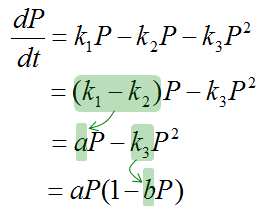DE - Modeling                                                                          Home : www.sharetechnote.com Population Model   This page will deal with how the population of a species would change over time. One thing you would notice from this page which may look a little bit different from physical models is about Governing Equation. In most of physical models, it would look obvious on why you have to use such an equation for the given model, but it may not look as such obvious in this kind of biological model. Most of the governing equation would be based on certain assumptions. If you change the assumption, you would have pretty different governing equation and as a result you will be end up with pretty different differential equation. So in case of biolological modeling, it is very important to understand clearly on what kind of assumptions are set for a specific case.     Population with No Death   The first case is the most simplest case. In this case, we assume that we see only a new birth of new individuals and there is no death in the population. Of course, there would be no such a case in real biological environment, but it would be beneficial to start with this ideal case since this is the simplest form and can be extendable to more realistic case just by adding some more factors (e.g, death, competition etc)   The Governing Law in this case can be described as below.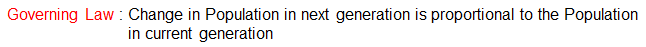If you convert the governing law into mathematical form as it is, it can be represented as shown below. (You may ask why the 'Change in Population' is proportional to P, not to P^2 nor P^3 etc. Your question is valid.. you can try with your imagination and check if your model can explain a real data)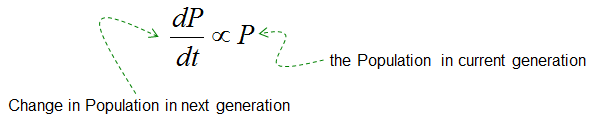If we introduce a proportional constant (let's call it k1), you can come out with an equation as shown below.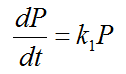Population with Death with Natural Cause   Now let's extend the previous model one step further to make it more realistic. In this mode, I would add one factor that would cause the decrease of the population. What would be the most common cause to decrease the population ? You know that there is no life that live forever. It would die off some time. so it would be natural to add the factor of death with natural cause (implying 'dying of age'). If we add this factor, the governing law can be as follows.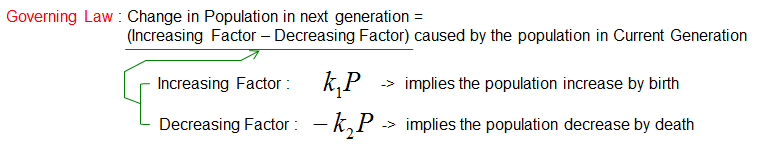If you just convert the Governing Law into mathematical form you would get the following equation. (You may ask the similar question as before, like 'why Death is proportional to P, not P^2, P^3 etc. Would the 'Decrease by Death' change if the cause of death changes ? like death by other factors like desease or epidemics ?. Very good questions. Think about it)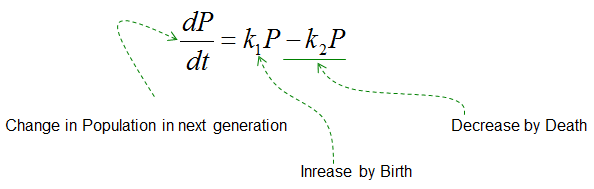I prefer to write the equation as above because you can understand the meaning of each terms more easily (almost intuitively). But many textbooks tend to write the equation in simplified form as below. In this case, it is important that the meaning of 'a' is the combination of two factors, i.e, net factors of birth and death.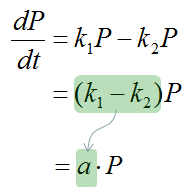Population with Death with Natural Cause and Competition   Now let's extend the previous model one step further. I would add another decreasing factor : Decreasing by Competition.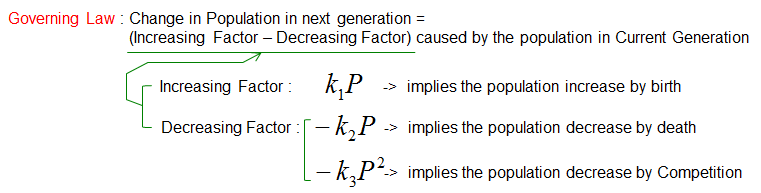If you just convert the Governing Law into mathematical form you would get the following equation. (You may ask the similar question as before, like 'why Competition is proportional to P^2, not P, P^3 etc.  Very good questions. Think about it. Some people say 'Competition is modeled as proportional to P^2 since normally competition usually happens when two individual encounter each other'. But it is also possible for you to come out with other competition model as well)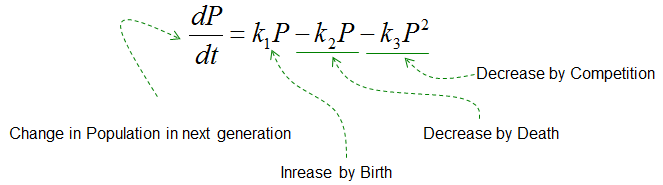Again, I prefer to write the equation as above because you can understand the meaning of each terms more easily (almost intuitively). But many textbooks tend to write the equation in simplified form as below.